CSE 5311 Section 501 Fall 1998
Homework 6
Due: April 15, 1998, in class

1. Run Dijkstra's algorithm on the directed graph shown in Figure 25.2 in the textbook, first using vertex s as the source and then using vertex y as the source. Show the d andvalues and the vertices in set S after each iteration of the while loop (this is Exercise 25.2-1 in the textbook).

```Iteration 0:
vertex =   1   2   3   4   5
d =   0 inf inf inf inf
pred = nil nil nil nil nil

Q = { 1 2 3 4 5 }

Iteration 1:
vertex =   1   2   3   4   5
d =   0   3 inf   5 inf
pred = nil   1 nil   1 nil

Q = { 2 3 4 5 }

Iteration 2:
vertex =   1   2   3   4   5
d =   0   3   9   5 inf
pred = nil   1   2   1 nil

Q = { 3 4 5 }

Iteration 3:
vertex =   1   2   3   4   5
d =   0   3   9   5  11
pred = nil   1   2   1   4

Q = { 3 5 }

Iteration 4:
vertex =   1   2   3   4   5
d =   0   3   9   5  11
pred = nil   1   2   1   4

Q = { 5 }

Iteration 5:
vertex =   1   2   3   4   5
d =   0   3   9   5  11
pred = nil   1   2   1   4

Q = { }

Iteration 0:
vertex =   1   2   3   4   5
d = inf inf inf inf   0
pred = nil nil nil nil nil

Q = { 1 2 3 4 5 }

Iteration 1:
vertex =   1   2   3   4   5
d =   3 inf   7 inf   0
pred =   5 nil   5 nil nil

Q = { 1 2 3 4 }

Iteration 2:
vertex =   1   2   3   4   5
d =   3   6   7   8   0
pred =   5   1   5   1 nil

Q = { 2 3 4 }

Iteration 3:
vertex =   1   2   3   4   5
d =   3   6   7   8   0
pred =   5   1   5   1 nil

Q = { 3 4 }

Iteration 4:
vertex =   1   2   3   4   5
d =   3   6   7   8   0
pred =   5   1   5   1 nil

Q = { 4 }

Iteration 5:
vertex =   1   2   3   4   5
d =   3   6   7   8   0
pred =   5   1   5   1 nil

Q = { }```
2. Run the Bellman-Ford algorithm on the directed graph shown in Figure 25.7 in the textbook using vertex y as the source. Relax edges in lexicographic order (see section 13-2 for a description of this concept) in each pass, and show the d andvalues after each pass. Now, change the weight of edge (y,v) to 4 and run the algorithm again, using z as the source (this is Exercise 25.3-1 in the textbook).

```Iteration 1:
vertex =   1   2   3   4   5
d =   2 998   7 inf   0
pred =   5   3   5 nil nil
Iteration 2:
vertex =   1   2   3   4   5
d =   2   5   6   9   0
pred =   5   3   4   1 nil
Iteration 3:
vertex =   1   2   3   4   5
d =   2   4   6   9   0
pred =   5   3   4   1 nil
Iteration 4:
vertex =   1   2   3   4   5
d =   2   4   6   9   0
pred =   5   3   4   1 nil

Iteration 1:
vertex =   1   2   3   4   5
d =   0   6   4   7   2
pred = nil   1   4   1   2
Iteration 2:
vertex =   1   2   3   4   5
d =   0   2   4   7   2
pred = nil   3   4   1   2
Iteration 3:
vertex =   1   2   3   4   5
d =   0   2   4   7  -2
pred = nil   3   4   1   2
Iteration 4:
vertex =   1   2   3   4   5
d =   0   2   4   7  -2
pred = nil   3   4   1   2

Iteration 1:
vertex =   1   2   3   4   5
d =   2 998   4 inf   0
pred =   5   3   5 nil nil
Iteration 2:
vertex =   1   2   3   4   5
d =   2   2   4   9   0
pred =   5   3   5   1 nil
Iteration 3:
vertex =   1   2   3   4   5
d =   0   2   2   9  -2
pred =   5   3   5   1   2
Iteration 4:
vertex =   1   2   3   4   5
d =   0   0   2   7  -2
pred =   5   3   5   1   2

Detected negative weight cycle.

Iteration 1:
vertex =   1   2   3   4   5
d =   0   6   4   7   2
pred = nil   1   4   1   2
Iteration 2:
vertex =   1   2   3   4   5
d =   0   2   4   7   2
pred = nil   3   4   1   2
Iteration 3:
vertex =   1   2   3   4   5
d =   0   2   2   7  -2
pred = nil   3   5   1   2
Iteration 4:
vertex =   1   2   3   4   5
d =   0   0   2   7  -2
pred = nil   3   5   1   2

Detected negative weight cycle.```
3. Given a weighted, directed graph G = (V, E) with no negative-weight cycles, let m be the maximum over all pairs of vertices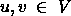of the minimum number of edges in a shortest path from u to v. In this case the shortest path is by weight, not by the number of edges. Suggest a change to the Bellman-ford algorithm that allows it to terminate in m+1 passes (this is Exercise 25.3-3 in the textbook).

The proof of Lemma 25.12 shows that for every v, d[v] has attained its final value after length(any shortest-weight path to v) iterations of Bellman-Ford. Thus after m passes, Bellman-Ford can terminate. We do not know m in advance, so we can not make the algorithm loop exactly m times and then terminate. However, if we make the algorithm stop when nothing changes any more, it will stop after m+1 iterations (after one iteration that makes no changes).

```New-Bellman-Ford(G, w, s)
Initialize-Single-Source(G,s)
changes = true
while changes = true
changes = false
for each edge (u,v) in E[G]
Relax-M(u,v,w)

Relax-M(u,v,w)
if d[v] > d[u] + w(u,v)
then d[v] = d[u] + w(u,v)
pi[v] = u
changes = true```

The test for a negative-weight cycle (based on the existence of a d value that would change if another relaxation step were done) has been removed above, because this version of the algorithm will never get out of the while loop unless all ds stop changing.

4. How can the output of the Floyd-Warshall algorithm be used to detect the presence of a negative-weight cycle? This is Exercise 26.2-5 in the textbook.

Here are two ways to detect negative-weight cycles:

1. Check the main-diagonal entries of the result matrix for a negative value. There is a negative-weight cycle iff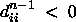for some vertex

• i:is a path weight from i to itself, so if it is negative, there is a path from i to itself (a cycle) with negative weight.
• If there is a negative-weight cycle, consider the one with the fewest vertices.

• If it has just one vertex, then some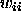< 0, so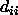starts out negative, and since d values are never increased, it is also negative when the algorithm terminates.
• If it has at least two vertices, let k be the highest-numbered vertex in the cycle, and let i be some other vertex in the cycle.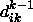and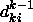have correct shortest-path weights, because they are not based on negative-weight cycles. (Neithernorcan include k as an intermediate vertex, and i and k are on the negative-weight cycle with the fewest vertices.) Since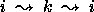is a negative-weight cycle, the sum of those two weights is negative, so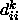will be set to a negative value. Since d values are never increased, it is also negative when the algorithm terminates.
• Alternatively, one could just run the normal Floyd-Warshall algorithm one extra iteration to see if any of the d values change. If there are negative cycles, then some shortest-path cost will be cheaper. If there are no such cycles, then no d-values will change because the algorithm gives the correct shortest paths.
5. Let G = (V,E) be a flow network with source s, sink t, and suppose each edge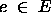has capacity c(e) = 1. Assume also, for convenience, that.

Suppose a maximum flow for G has been computed, and a new edge with capacity 1 is added to E. Describe how the maximum flow can be efficiently updated. It is not the value of the flow that must be updated, but the flow itself. Analyze the run time of your algorithm.

Execute one iteration of the Ford-Fulkerson algorithm. The new edge in E adds a new edge to the residual graph, so look for an augmenting path and update the flow if a path is found.

Time: O(V + E) = O(E) if a path is found with depth-first or breadth-first search.

Only 1 iteration is needed because adding the capacity-1 edge increases the capacity of the min cut by at most 1. Thus the max flow (equal to the min cut capacity) increases by at most 1. Since all edge capacities are 1, any augmentation increases flow by 1, so only 1 augmentation can be needed.

6. Consider a parallel version of the Bellman-Ford algorithm (serial pseudocode shown below) where the for loops beginning at lines 3 and 5 are executed in parallel (i.e., constant time).

```Initialize-Single-Source(G,s)
for  each vertex v in V[G]
d[v] = infinity
pi[v] = nil
d[s] = 0
Relax(u,v,w)
if d[v] > d[u] + w(u,v)
then d[v] = d[u] + w(u,v)
pi[v] = u

Bellman-Ford(G,w,s)
1.    Initialize-Single-Source(G,s)
2.    for i = 1 to |V[G]| - 1
3.       for each edge (u,v) in E[G]
4.          Relax(u,v,w)
5.       for each edge (u,v) in E[G]
6.          if d[v] > d[u] + w(u,v)
7.          then return {\sc false}
8.    return true```

What is the running time, the speedup, and the efficiency of the parallel algorithm?

The serial run time of the Bellman-Ford algorithm is O(VE). If the loops are run in parallel and the number of processors P is at least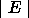, then the parallel algorithm runs in time O(V), which results in a speedup S of O(VE)/O(V) = E and an efficiency E of V/P.

Diane J. Cook
Mon Feb 2 22:01:07 CST 1998